# Demystifying Class Imbalance in Datasets – with R# Class Imbalance

Class Imbalance is when the prediction/Target classes are largely skewed, or few of the classes have distinctly lower sample size than the dominant prediction classes.

## Data Understanding

Here we are using Kaggle data to explain class imbalance and explain how to handle it. Here is the link to data , we are using  Credit card fraud detection.

Let’s have a look to our data.

```data <- read_csv("../input/creditcard.csv")
```Let’s have a look to our target column

```prop.table(table(data\$Class))
```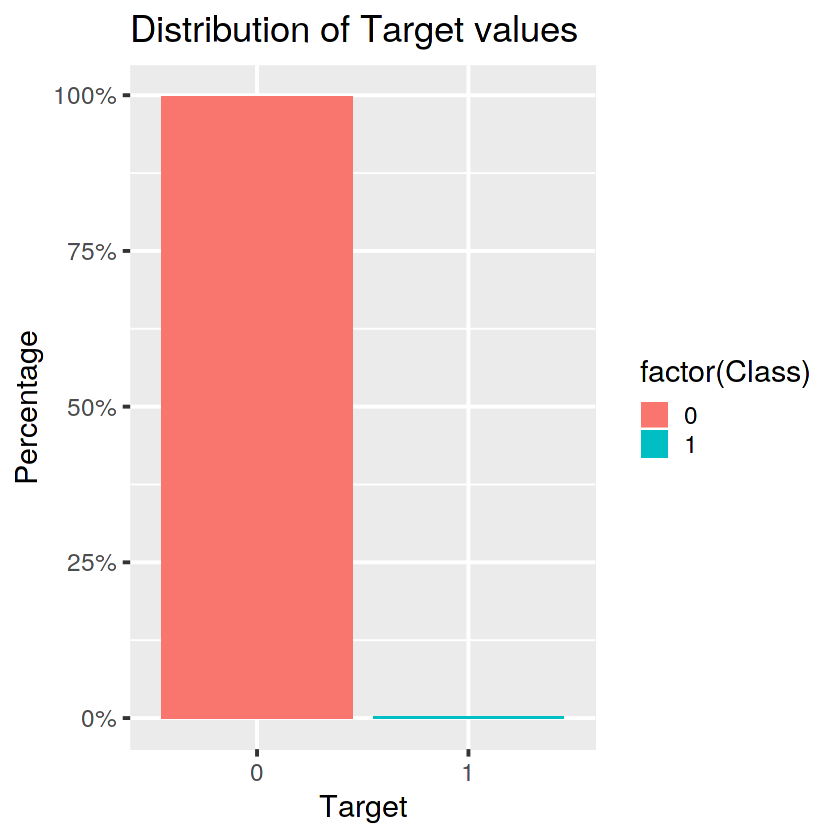We can see there is a huge class imbalance in this dataset. We have approximately 1% of the positive cases and about 99% of negative cases. In this scenario, our model will be biased towards the majority class (Target = 0 ). Our model will have good accuracy for majority class but will have poor accuracy for minority class(Target = 1).

## Different Classification Model

We have approach to different classification techniques and compare them to select best model for our prediction and reason to select the model.

• Linear Classification model
• Tree based model

### Linear Classification

Linear classification models are usually not great at handling class imbalance in dataset. We will verify our theory by running a linear classification model on our dataset.

`data_glm <- glm(Class ~ ., data = train, family = binomial(link = 'logit'))`

#### Variable ImportanceComputing the variable importance from linear classification model. Following are the most important features from this model.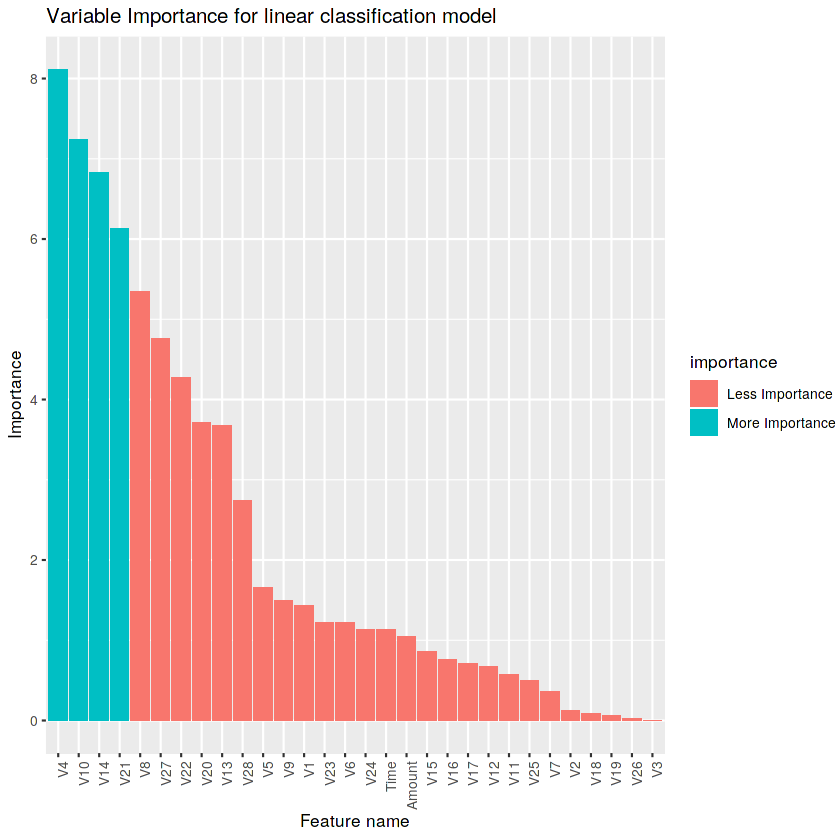We can see the overall variable importance across all the features.

#### Model Prediction

Let’s have a look to confusion matrix to see the accuracy of our model.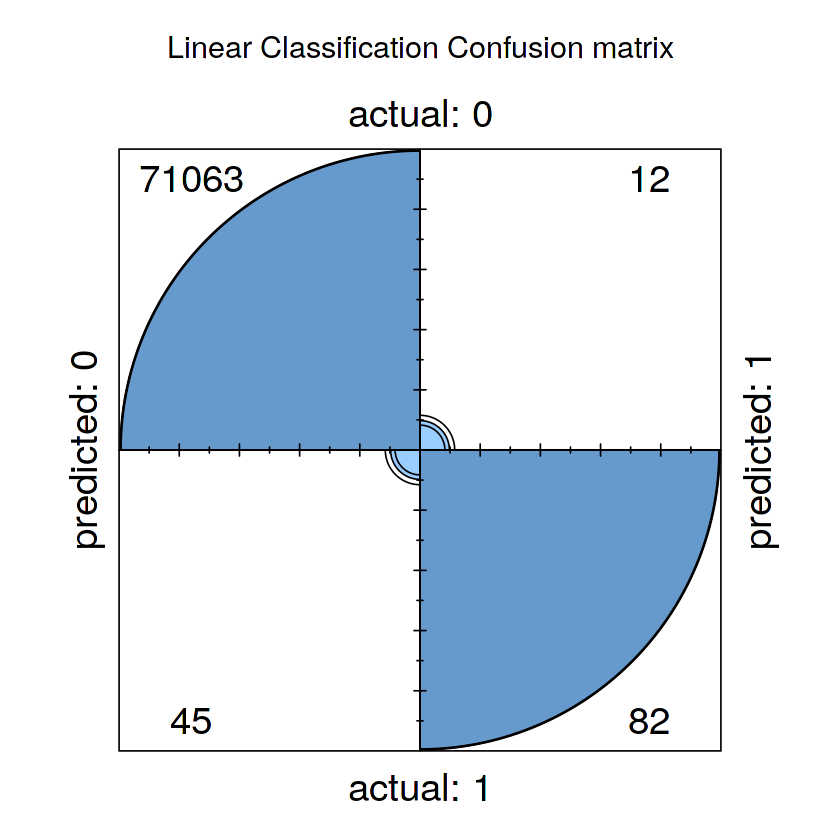Looking at the confusion matrix we can see that the model made a lot of false positive prediction. Our model is biased towards the positive class i.e. the majority class.We can find some interesting insights from these metrics. We can see Precision = 0.8723404. Higher precision value shows that  there are fewer false positives. We can see Recall =0.6456693 which is low and indicates that we have higher number of false negatives. We also observed F1 =0.7420814 which is also low. Lower F1 score suggests weak accuracy of the model.

ROC-AUC still gives a decent score to our linear model. Therefore it is not a good indicator of its performance.

### Tree Based Classification

Now we will run a tree based model on the same dataset. We are choosing Random Forest for this task as it is much  better at handling class imbalance.

#### Model Prediction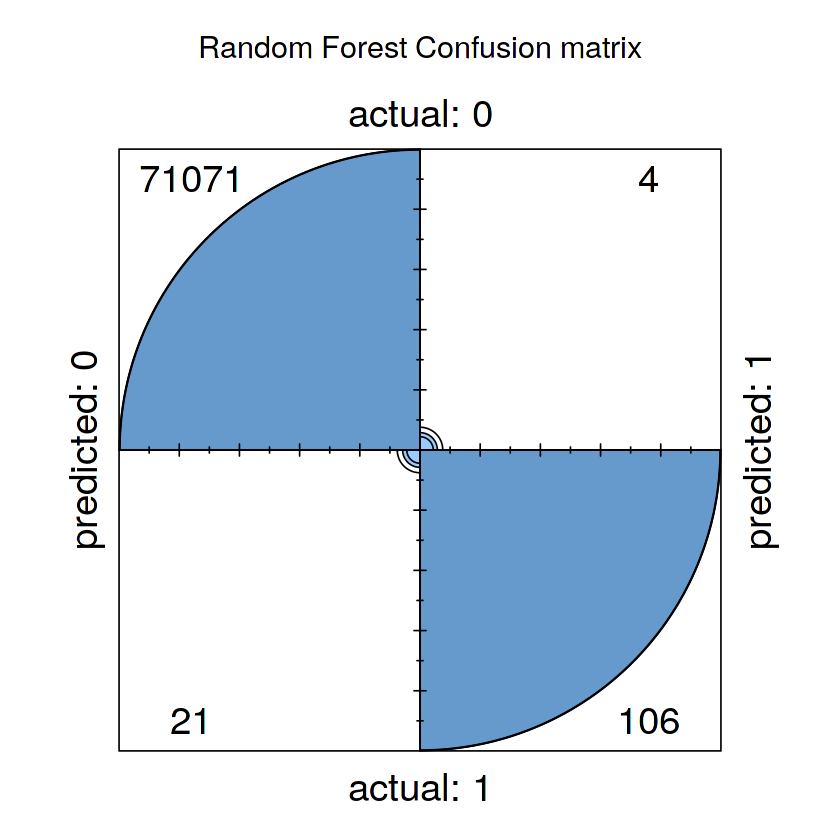Looking at the confusion matrix we can see that the model made fewer false positive and false negative predictions compared to the linear classification model.

## Comparison of Models

Here we will compared the models with different metrics score.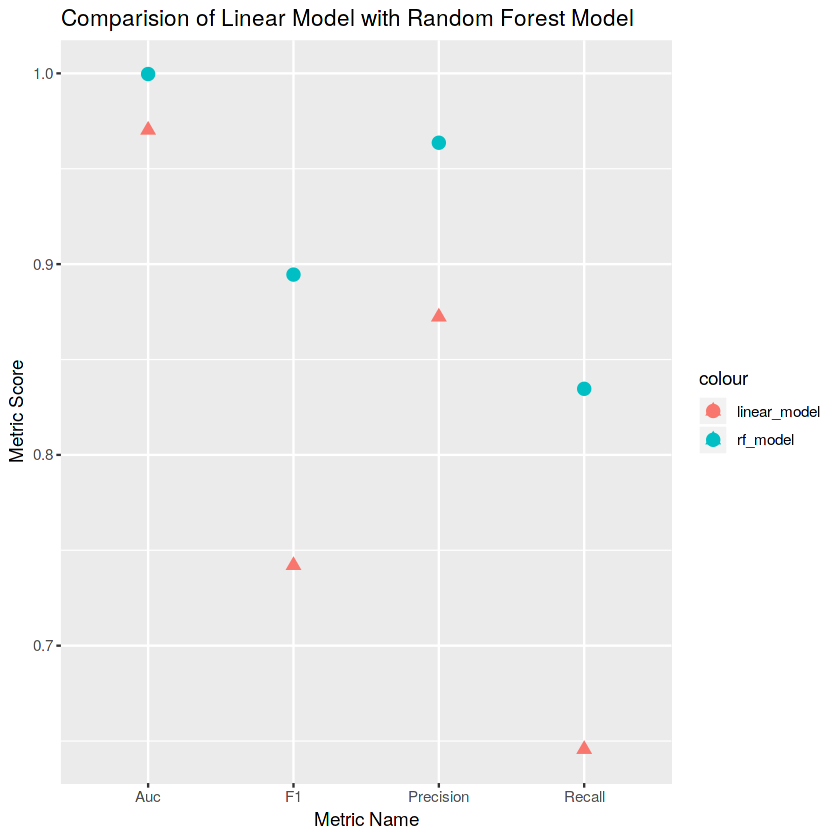### Precision

We can see higher precision value for Random forest model which shows that  there are fewer false positives in Random forest compared to Linear model.

### Recall

We can see higher Recall value for Random forest model which indicates that we have lower number of false negatives  in Random forest compared to Linear model.

### F1 Score

We also observe F1 value of Random Forest higher than linear classification model. Higher F1 score suggests strong accuracy of the model.
Based on our comparison we can see that Random Forest is best for handling Class imbalance problem.

#### Most suitable Metric

F1 is the harmonic mean of recall and precision. So, since we have a small positive class, then F1 score makes more sense. For this task, we are not using AUC because it’s not measuring class imbalance correctly.

So far we saw that the tree based models are best suited for class imbalance. There are other techniques to handle class imbalance as well. We can resample our training dataset to create balanced dataset. There are two ways to resample training data i.e; Under sampling and Over sampling.

# Under Sampling

Under sampling is used when the amount of collected data is sufficient.  It may be used to remove some of the majority classes for a more balanced amount of positive results in training but it may potentially discard some useful or important data. The most common technique is known as Cluster centroid.

### Over Sampling

Over sampling is used when the amount of data collected is insufficient. It maybe used to duplicate some of the minority classes for a more balanced amount of positive results in training.   The most common technique is known as SMOTE: Synthetic Minority Over-sampling Technique.